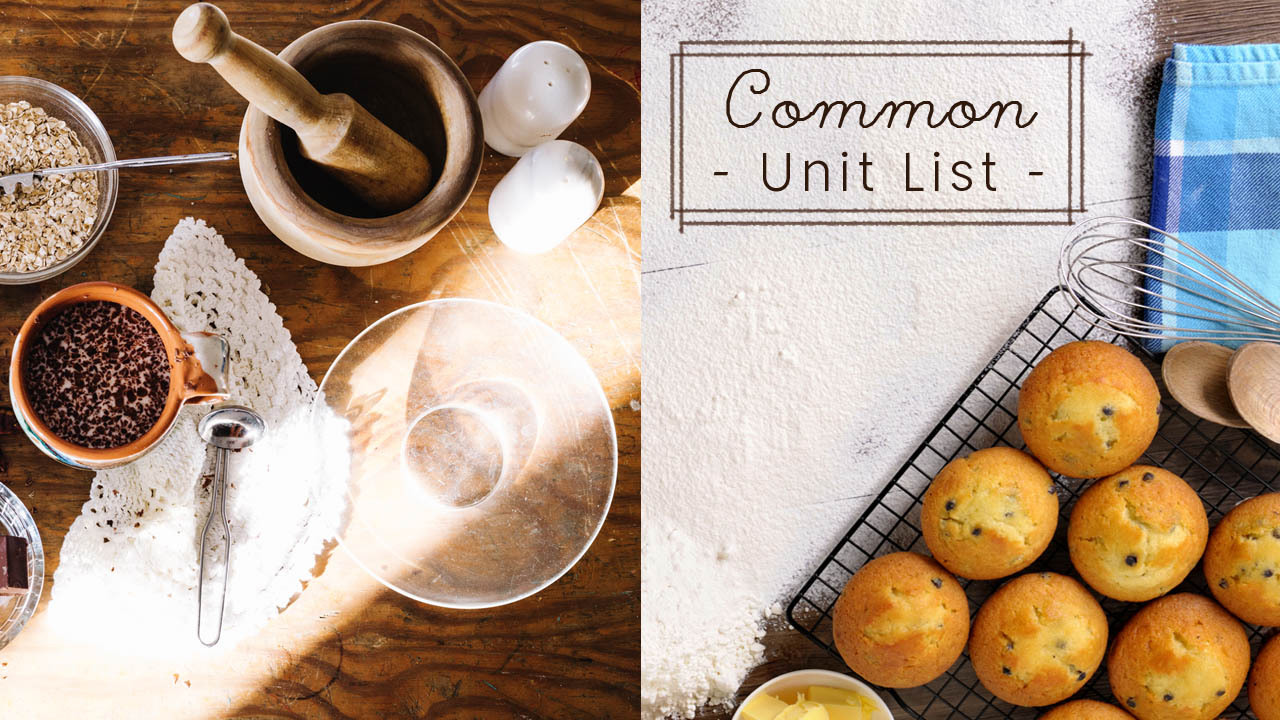｜小叮嚀｜

【溫度換算】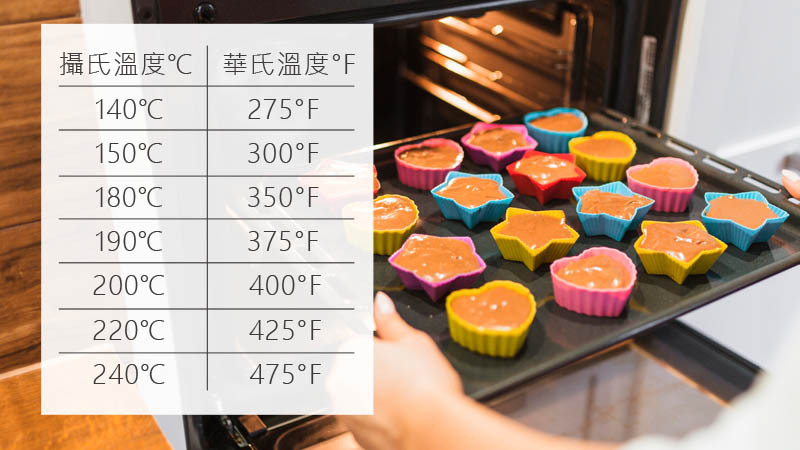(華氏-32)*5/9=攝氏

【常見量匙份量】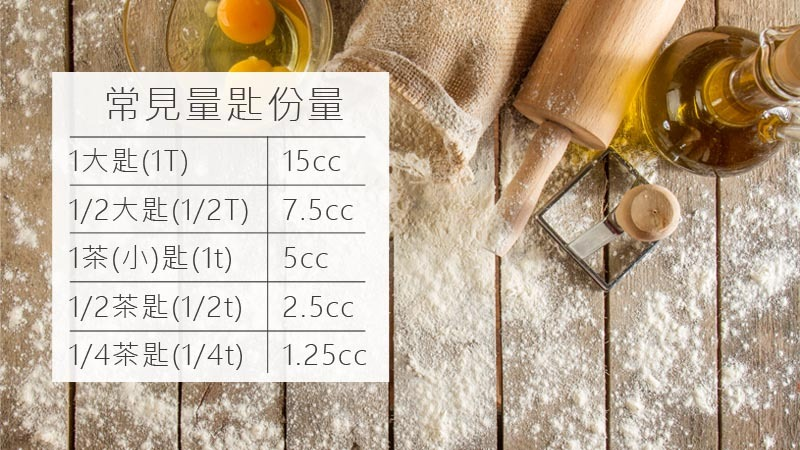• 1大匙(1T)=15cc
• 1/2大匙(1/2T)=7.5cc
• 1茶(小)匙(1t)=5cc
• 1/2茶匙(1/2t)=2.5cc
• 1/4茶匙(1/4t)=1.25cc

【容量換算】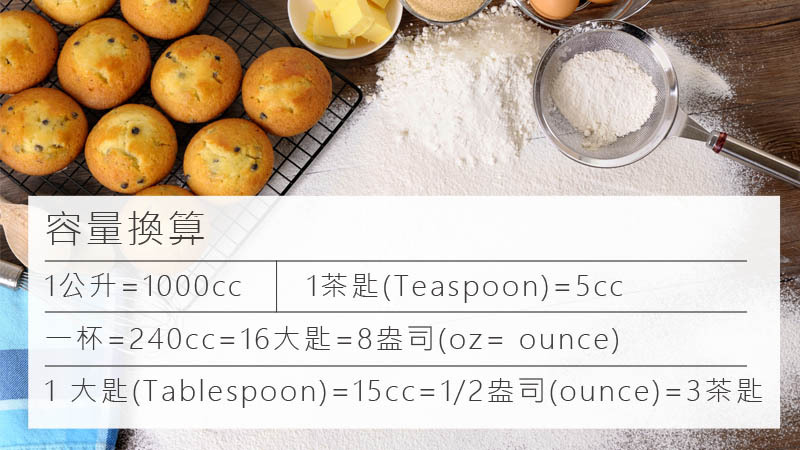• 1公升=1000cc
• 一杯=240cc=16大匙=8盎司(oz= ounce)1 大匙(Tablespoon)=15cc=1/2盎司(ounce)=3茶匙
• 1茶匙(Teaspoon)=5cc
• 2杯=480cc=16盎司(ounce)=1品脫(pt)

｜小知識｜

【重量換算】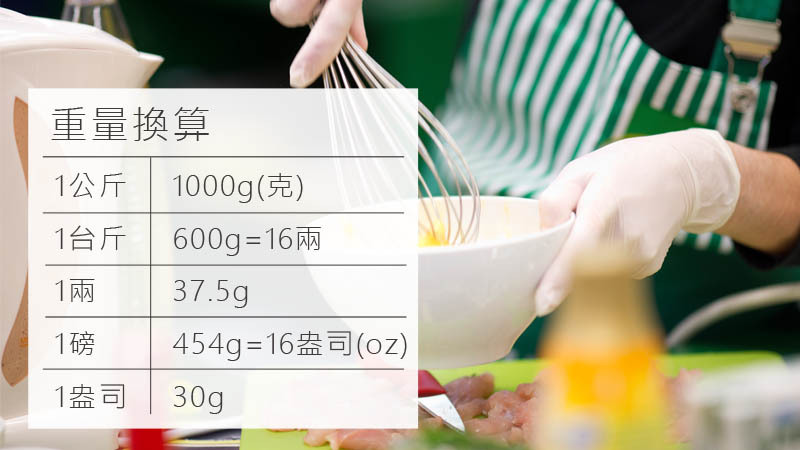• 1 kg(公斤)=1000g(克)
• 1台斤=600g=16兩
• 1 兩=37.5g
• 1 磅=454g=16盎司(oz)=1品脫(pint)
• 1盎司(oz)=30g

【其他常用食材換算表】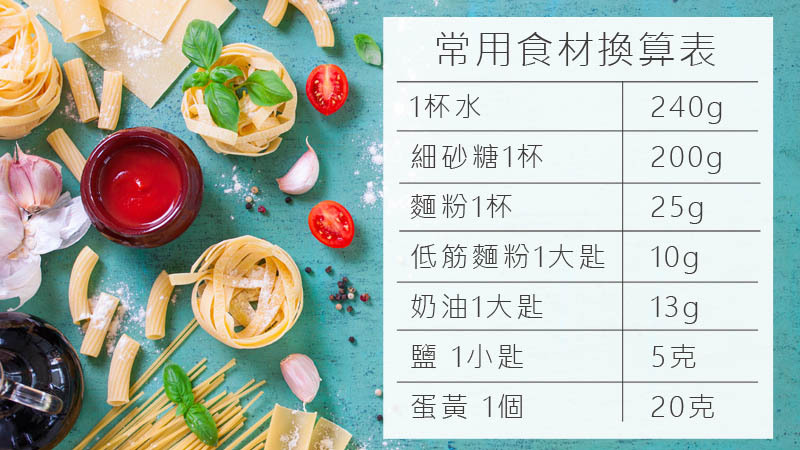• 1杯水=240g =240cc
• 細砂糖1杯=200g
• 麵粉1杯=125g

• 蜂蜜1大匙=22g
• 低筋麵粉1大匙=10g
• 奶油1大匙=13g
• 鹽 1小匙﹦5克
• 小蘇打 1小匙﹦4.7克

• 蛋黃 1個﹦20克
• 蛋白 1個﹦30克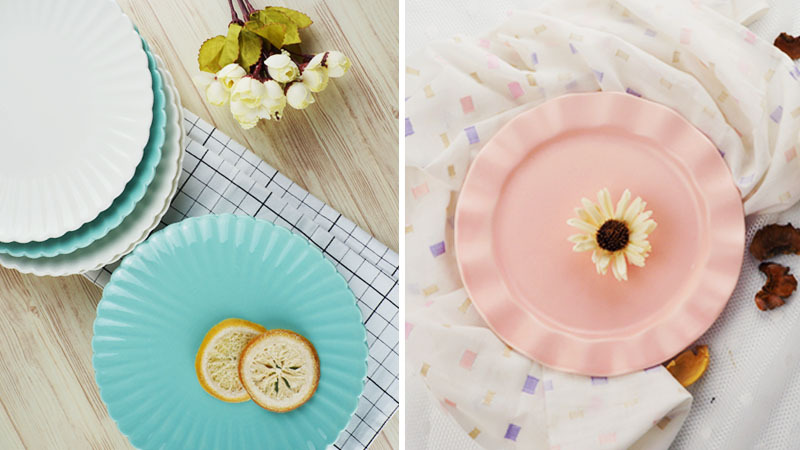✏【延伸閱讀，這些文章你也會有興趣】
1. 微波爐的使用禁忌！這5種常見物品千萬別放入加熱
2. 馬克杯細菌藏在哪？這些清洗技巧一般人都不會注意
3. 讀過了這篇檸檬使用大全，你不會再浪費任一滴檸檬汁
4. 消除炎炎夏日，簡單又健康的自製飲品
5. 便當擺盤小撇步，三個小技巧升級便當顏值